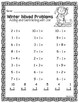Subject
Resource Type
File Type

PDF

(8 MB|71 pages)
Also included in:
1. This is an awesome BUNDLE for addition and subtraction practice. It includes pages for adding and subtracting specific numbers, adding and subtracting using number lines, adding and subtracting using ten frames, task cards, and write the room pages. Each activity includes a fun Winter theme.Also T
\$21.00
\$14.70
Save \$6.30
Product Description

This is an awesome product for addition and subtraction practice. It includes worksheets adding and subtracting specific numbers, adding and subtracting using number lines, adding and subtracting using ten frames, task cards, and write the room pages. Each activity includes a fun Winter theme.

Also Try:

Winter Color By Code Bundle: Winter, Christmas, and Valentine's Day

Winter Color By Code Adding and Subtracting Using a Number Line

Fall Subtraction Practice

Fall Color By Code: Adding Using A Number Line

This product includes the following:

Adding and Subtracting Using a 0-10 Number Line (2 pages)

Adding and Subtracting Using a 0-20 Number Line (2 pages)

Adding and Subtracting Using 1 Ten Frame (2 pages)

Adding and Subtracting Using 2 Ten Frames (2 pages)

Adding and Subtracting within 10 (2 pages)

Adding and Subtracting within 20 (2 pages)

Adding and Subtracting with Fact Families (Add and Subtract within 10)(2 pages)

Adding and Subtracting with Fact Families (Add and Subtract within 20)(2 pages)

Write the Room (Add and Subtract within 10)

Write the Room (Add and Subtract within 20)

Total Pages
71 pages
Included
Teaching Duration
N/A
Report this Resource to TpT
Reported resources will be reviewed by our team. Report this resource to let us know if this resource violates TpT’s content guidelines.